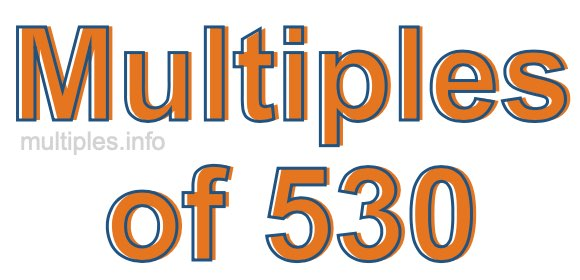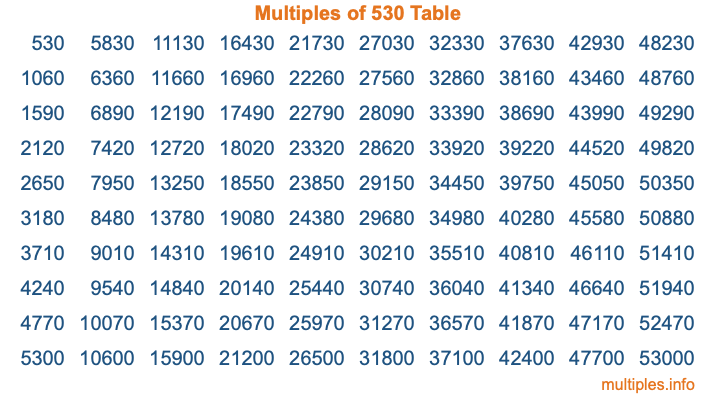Multiples of 530Welcome to the Multiples of 530 page. Here we will first teach you everything you will ever need to know about the multiples of 530, and then give you a study guide summary of everything we taught you to make sure you remember it all. Use this page to look up facts and learn information about the multiples of 530. This page will make you a multiples of five hundred thirty expert!

Definition of Multiples of 530
Multiples of 530 are all the numbers that when divided by 530 equal an integer. Each of the multiples of 530 are called a multiple. A multiple of 530 is created by multiplying 530 by an integer.

Therefore, to create a list of multiples of 530, you start with 1 multiplied by 530, then 2 multiplied by 530, then 3 multiplied by 530, and so on for as long as you want. Thus, the list of the first five multiples of 530 is 530, 1060, 1590, 2120, and 2650. To see a larger list of multiples of 530, see the printable image of Multiples of 530 further down on this page. We also have a category where you can choose any nth multiple of 530.

Multiples of 530 Checker
The Multiples of 530 Checker below checks to see if any number of your choice is a multiple of 530. In other words, it checks to see if there is any number (integer) that when multiplied by 530 will equal your number. To do that, we divide your number by 530. If the the quotient is an integer, then your number is a multiple of 530.

Is  a multiple of 530?

Least Common Multiple of 530 and ...
A Least Common Multiple (LCM) is the lowest multiple that two or more numbers have in common. This is also called the smallest common multiple or lowest common multiple and is useful to know when you are adding our subtracting fractions. Enter one or more numbers below (530 is already entered) to find the LCM.

Check out our LCM Calculator if you need more details about the Least Common Multiple or if you need the LCM for different numbers for adding and subtraction fractions.

nth Multiple of 530
As we stated above, 530 is the first multiple of 530, 1060 is the second multiple of 530, 1590 is the third multiple of 530, and so on. Enter a number below to find the nth multiple of 530.

th multiple of 530

Multiples of 530 vs Factors of 530
530 is a multiple of 530 and a factor of 530, but that is where the similarities end. All postive multiples of 530 are 530 or greater than 530. All positive factors of 530 are 530 or less than 530.

Below is the beginning list of multiples of 530 and the factors of 530 so you can compare:

Multiples of 530: 530, 1060, 1590, 2120, 2650, etc.

Factors of 530: 1, 2, 5, 10, 53, 106, 265, 530

As you can see, the multiples of 530 are all the numbers that you can divide by 530 to get a whole number. The factors of 530, on the other hand, are all the whole numbers that you can multiply by another whole number to get 530.

It's also interesting to note that if a number (x) is a factor of 530, then 530 will also be a multiple of that number (x).

Multiples of 530 vs Divisors of 530
The divisors of 530 are all the integers that 530 can be divided by evenly. Below is a list of the divisors of 530.

Divisors of 530: 1, 2, 5, 10, 53, 106, 265, 530

The interesting thing to note here is that if you take any multiple of 530 and divide it by a divisor of 530, you will see that the quotient is an integer.

Multiples of 530 Table
Below is an image of the first 100 multiples of 530 in a table. The table is in chronological order, column by column. The first column has the first ten multiples of 530, the second column has the next ten multiples of 530, and so on.The Multiples of 530 Table is also referred to as the 530 Times Table or Times Table of 530. You are welcome to print out our table for your studies.

Negative Multiples of 530
Although not often discussed or needed in math, it is worth mentioning that you can make a list of negative multiples of 530 by multiplying 530 by -1, then by -2, then by -3, and so on, to get the following list of negative multiples of 530:

-530, -1060, -1590, -2120, -2650, etc.

Multiples of 530 Summary
Below is a summary of important Multiples of 530 facts that we have discussed on this page. To retain the knowledge on this page, we recommend that you read through the summary and explain to yourself or a study partner why they hold true.

There are an infinite number of multiples of 530.

A multiple of 530 divided by 530 will equal a whole number.

530 divided by a factor of 530 equals a divisor of 530.

The nth multiple of 530 is n times 530.

The largest factor of 530 is equal to the first positive multiple of 530.

530 is a multiple of every factor of 530.

530 is a multiple of 530.

A multiple of 530 divided by a divisor of 530 equals an integer.

530 divided by a divisor of 530 equals a factor of 530.

Any integer times 530 will equal a multiple of 530.

Multiples of a Number
Here you can get the multiples of another number, all with the same attention to detail as we did for multiples of 530 on this page.

Multiples of
Multiples of 531
Did you find our page about multiples of five hundred thirty educational? Do you want more knowledge? Check out the multiples of the next number on our list!